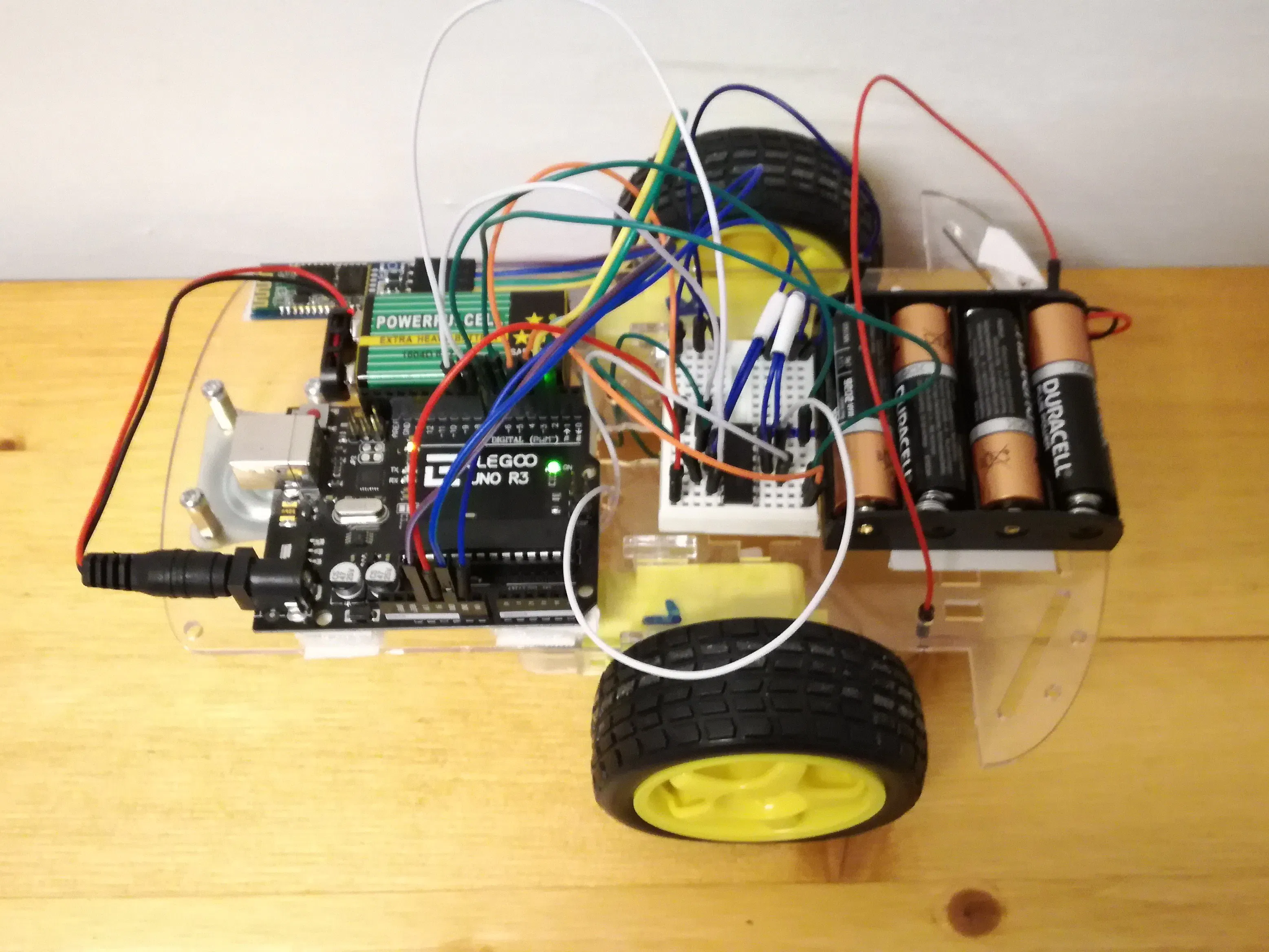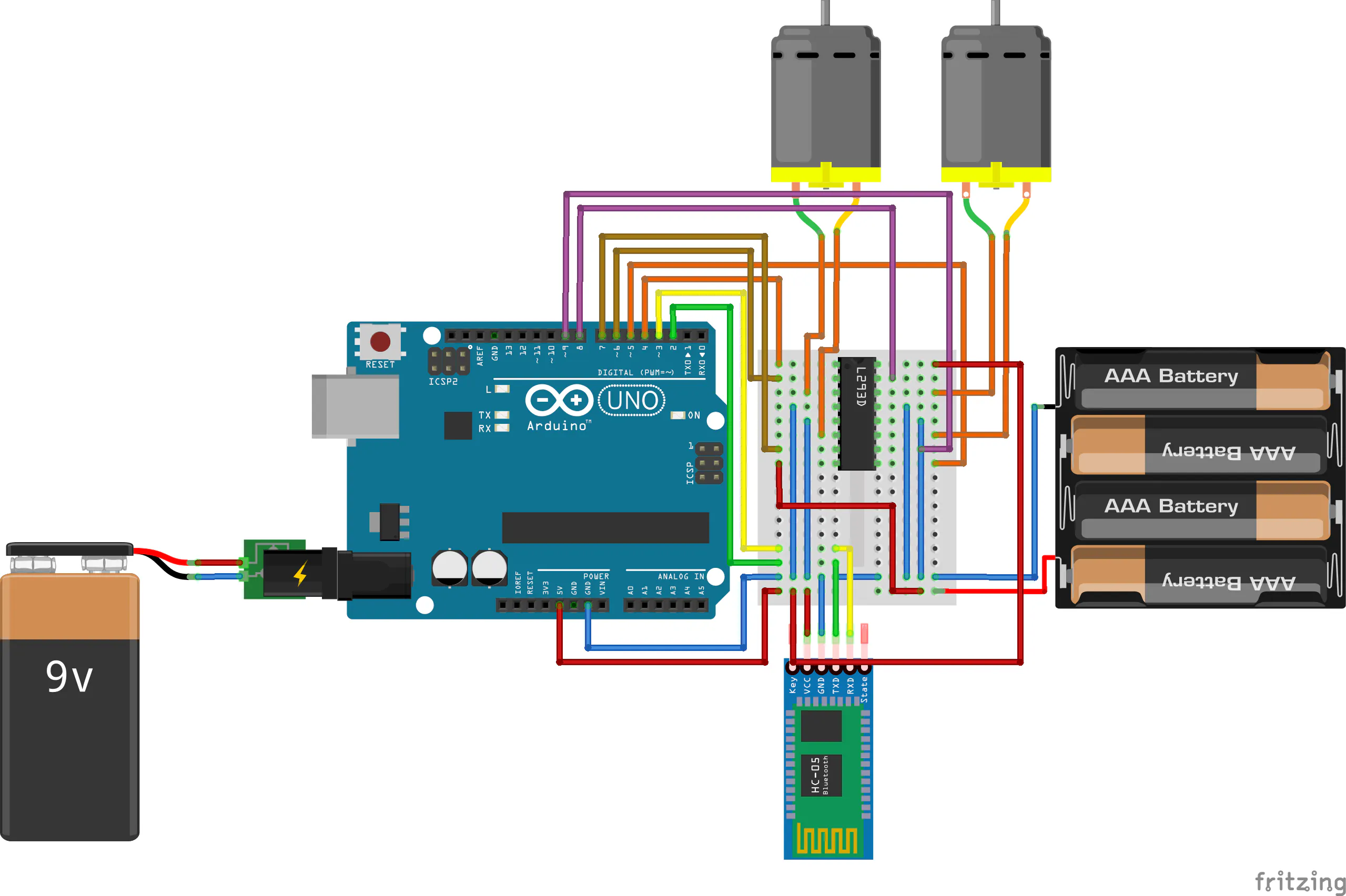# Guide Your Car Model Remotely with Bluetooth

Project for creating a car model guided remotely over Bluetooth directly from your smartphone.

IntermediateShowcase (no instructions)506## Things used in this project

### Hardware componentsArduino UNO & Genuino UNO
×1

### Software apps and online servicesArduino IDE
 Bluetroller, serial bluetooth controller

## Schematics

### Main electronic schema

This is the main schema for the project.## Code

### Main code of the project

Arduino
This is the code for elaborating the bluetooth commands and to control the motors from the bluetooth device. For details see the project description.
```SoftwareSerial bluetooth(BLE_INPUT, BLE_OUTPUT);
String command = "";
int angle = 0;
int power_percentage = 0;
const float maxPower = 255.0;
float speedLimit;
float normAngle;
void setup() {
bluetooth.begin(9600);
}
void loop() {
speedLimit = (float(power_percentage) / 100) * maxPower;
if (angle > 180 && angle < 360) {
setDirectionClockwise(false);
} else  {
setDirectionClockwise(true);
}
// L MOTOR
if (angle <= 90 || angle >= 270) {
analogWrite(MOTOR_L_POW, int(speedLimit));
} else if (angle >= 180 && angle < 270) {
normAngle = angle % 90;
analogWrite(MOTOR_L_POW, int((normAngle * speedLimit) / 90));
} else {
normAngle = (180 - angle) % 90;
analogWrite(MOTOR_L_POW, int((normAngle * speedLimit) / 90));
}
// R MOTOR
if (angle >= 90 && angle <= 270) {
analogWrite(MOTOR_R_POW, int(speedLimit));
} else if (angle > 270) {
normAngle = (180 - angle) % 90;
analogWrite(MOTOR_R_POW, int((normAngle * speedLimit) / 90));
} else {
normAngle = (angle) % 90;
analogWrite(MOTOR_R_POW, int((normAngle * speedLimit) / 90));
}
}
void setDirectionClockwise(boolean clockwise) {
if (clockwise) {
digitalWrite(MOTOR_L_CTRL1, HIGH);
digitalWrite(MOTOR_L_CTRL2, LOW);
digitalWrite(MOTOR_R_CTRL1, HIGH);
digitalWrite(MOTOR_R_CTRL2, LOW);
} else {
digitalWrite(MOTOR_L_CTRL1, LOW);
digitalWrite(MOTOR_L_CTRL2, HIGH);
digitalWrite(MOTOR_R_CTRL1, LOW);
digitalWrite(MOTOR_R_CTRL2, HIGH);
}
}
if (bluetooth.available()) {
char currentChar;
while (true) {
if (currentChar == START_SYMBOL) {
command = "";
} else if (currentChar == MIDDLE_SYMBOL) {
angle = command.toInt();
command = "";
} else if (currentChar == END_SYMBOL) {
power_percentage = command.toInt();
command = "";
break;
}  else {
command += currentChar;
}
}
command = "";
}
}
```